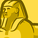# New to Qlik Sense

If you’re new to Qlik Sense, start with this Discussion Board and get up-to-speed quickly.

Announcements
cancel
Showing results for
Did you mean:Contributor III

## Year to year comparison of cumulated quarter values

Hello Everyone!

What I need to do is either to create a marker or a set analysis in Qlik Sense that does the following automatically:Displayed should be the last completed Quarter, offset is the first quarter of the year the last quarter displayed is in (and the same quarters in PY).

For example today, the 4th of January 2022, displayed should be the cumulative values of Q1 - Q4 2021 vs. Q1 - Q4 2020.

However, at the beginning of Q2 2022 it should switch to Q1 2021 vs. Q1 2022.

Beginning of Q3 2022, Q1 - Q2 2022 vs. Q1 - Q2 2022 and so forth...

So far all my efforts and searches where to no avail.

Does someone have an idea how to solve this riddle (would a date field QuarterYear or YearQuarter help?)?

Kind regards

Stefan

Labels (3)

• ### Set Analysis

1 Solution

Accepted SolutionsContributor III
Author

We seem to have solved it like this, if the report for Q4 is  generated in January the following year:

Actual Year

if(ceil(month(today()))< 2, sum({<[Year]= {'\$(=max([Year])-1)'}>} [KPI] )
, sum({<[Year]= {'\$(=max([Year]))'}, [Date] = {'<=\$(=QuarterEnd(today())-1)'}>} [KPI]))

Previous Year

if(ceil(month(today()))< 2, sum({<[Year]= {'\$(=max([Year])-2)'}>} [KPI])
, sum({<[Year]= {'\$(=max([Year])-1)'}, [Date] = {'<=\$(=QuarterEnd(today())-1)'}>} [KPI]))Contributor III
Author

We seem to have solved it like this, if the report for Q4 is  generated in January the following year:

Actual Year

if(ceil(month(today()))< 2, sum({<[Year]= {'\$(=max([Year])-1)'}>} [KPI] )
, sum({<[Year]= {'\$(=max([Year]))'}, [Date] = {'<=\$(=QuarterEnd(today())-1)'}>} [KPI]))

Previous Year

if(ceil(month(today()))< 2, sum({<[Year]= {'\$(=max([Year])-2)'}>} [KPI])
, sum({<[Year]= {'\$(=max([Year])-1)'}, [Date] = {'<=\$(=QuarterEnd(today())-1)'}>} [KPI]))